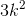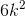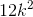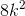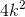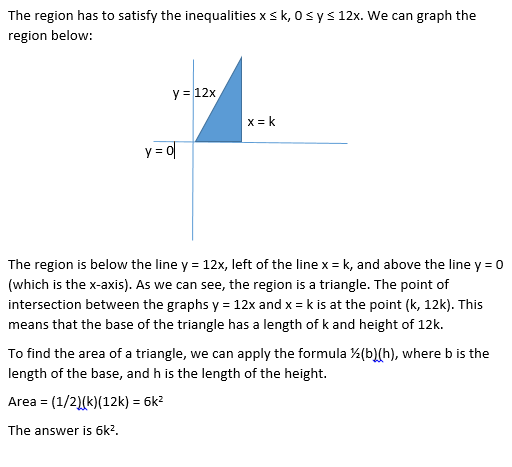# Advanced Geometry : How to graph a two-step inequality

## Example Questions

### Example Question #1 : How To Graph A Two Step Inequality

Solve and graph the following inequality: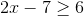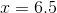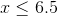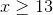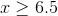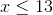Explanation:

To solve the inequality, the first step is to addto both sides: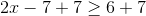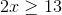The second step is to divide both sides by: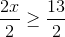To graph the inequality, you draw a straight number line. Fill in the numbers fromto infinity. Infinity can be designated by a ray. Be sure to fill in the number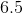, since the equation indicated greater than OR equal to.

The graph should look like:### Example Question #2 : How To Graph A Two Step Inequality

Points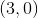and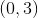lie on a circle. Which of the following could be the equation of that circle?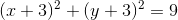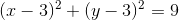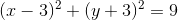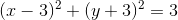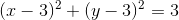Explanation:

If we plug the pointsandinto each equation, we find that these points work only in the equation. This circle has a radius ofand is centered at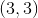.

### Example Question #3 : How To Graph A Two Step Inequality

Which of the following lines is perpendicular to the line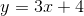?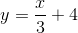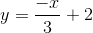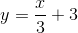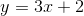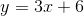Explanation:

The key here is to look for the line whose slope is the negative reciprocal of the original slope.

In this case,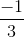is the negative reciprocal of.

Therefore, the equation of the line which is perpendicular to the original equation is:### Example Question #201 : Graphing

Which inequality does this graph represent?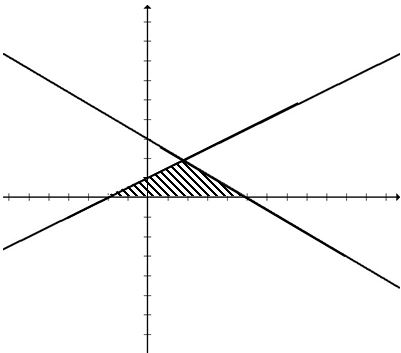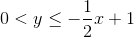;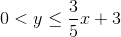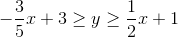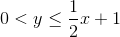;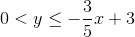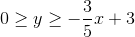;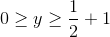;Explanation:

The two lines represented are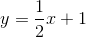and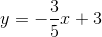. The shaded region is below both lines but above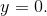### Example Question #202 : Graphing

What is the area of the shaded region for the following inequality: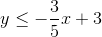;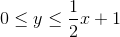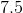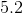Explanation:

This inequality will produce the following graph:The shaded area is a triangle with base 7 and height 2.

To find the area, plug these values into the area formula for a triangle,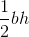.

In this case, we are evaluating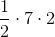, which equals 7.

### Example Question #203 : Graphing

What is the area of the shaded region for this system of inequalities: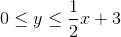;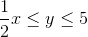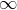Explanation:

Once graphed, the inequality will look like this: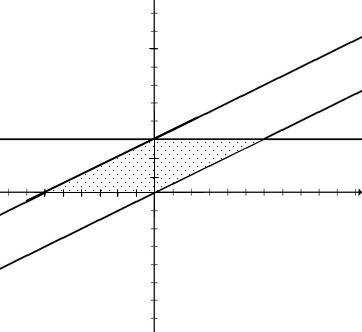To find the area, it is easiest to consider it as 2 congruent triangles with base 6 and height 3.

The total area will then be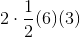, or just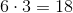.

### Example Question #204 : Graphing

Find the-intercept for the following: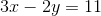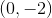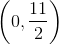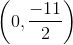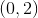Explanation: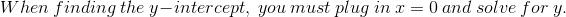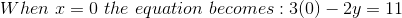.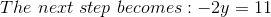.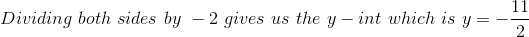.

### Example Question #1 : Graphing

Let D be the region on the (x,y) coordinate plane that contains the solutions to the following inequalities: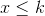, whereis a positive constant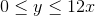Which of the following expressions, in terms of, is equivalent to the area of D?